# Graph Widget

The graph widget displays the topology and relationships of servers, virtual machines, and managers with their corresponding status.

Series Mappings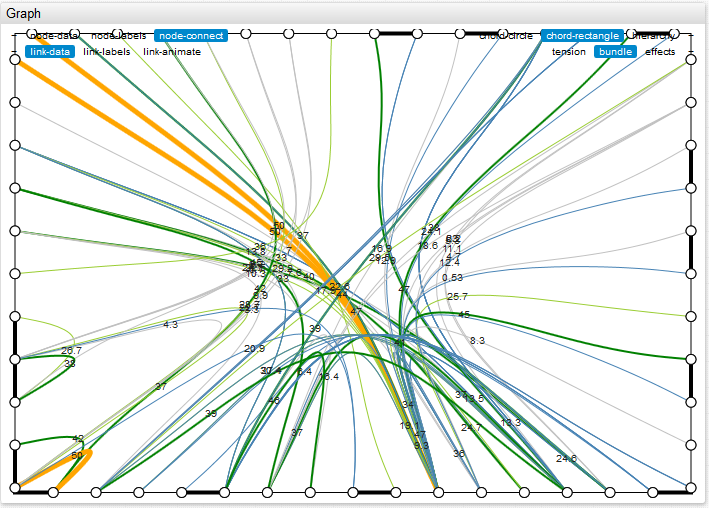Chart Lab: View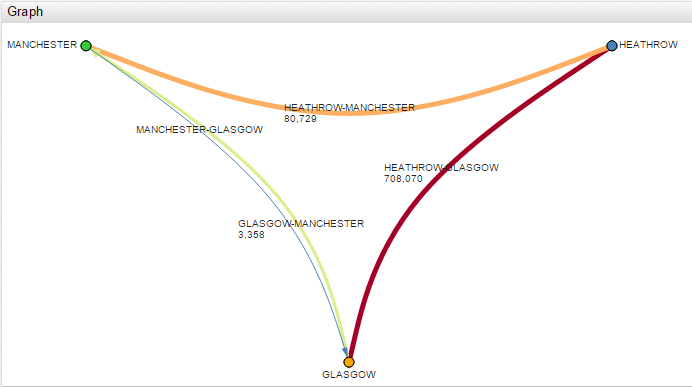#### Structure

The structure of the graph is given by the description of its vertices and edges. The [node] tag is used to describe each vertex, and the hierarchy of vertices is determined by the parent parameter, which sets the predecessor. The [link] tag is used to describe the edges. Adjacent edges, with vertices, are determined by the [node] tag.

Time Series can be linked to edges or vertices by indicating the alias in the series parameter of [link] or [node] tags. Also, you can list the loaded edges or vertices in the links or nodes of the [series] parameter.

##### Example:
```[widget] type = graph title = Channel_Graph alert-expression = value > 100000 alert-style = stroke: red bundle = false circle = false tension = 0 [node] id = queue_1   [node] id = queue_2   [node] id = queue_3   [link] id = channel_1 nodes = queue_1-queue_2 series = ch1   [link] id = channel_2 nodes = queue_2->queue_3   [link] id = channel_3 nodes = queue_1<-queue_3   [series] entity = gr01 metric = message_count links = channel_2   [tag] name = channel_name value = channel2   [series] alias = ch1 entity = gr01 metric = message_count   [tag] name = channel_name value = channel1```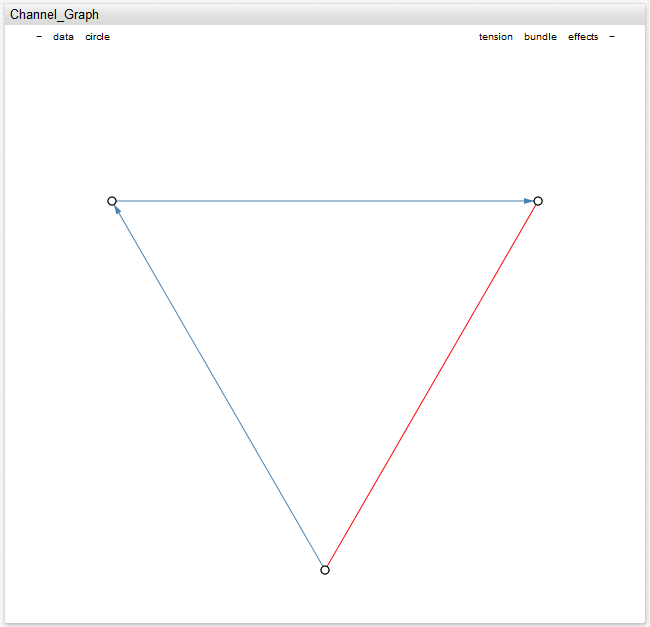#### Hierarchical Mode

To activate the hierarchical mode use the mode setting:

`mode = hierarchy`

In the event of an alert-expression response on the time series of the vertex or edge to which the time series is tied. Value of alert-style can be either string or script. If alert-style is script, the variable `alert` is available, and it is equal to the value that the alert returns. The following styles are applied (in a specified order) in the parameters below:

For example:

`link-alert-style = if (alert > 99) return 'stroke-width:3px';`
`node-alert-style = if (alert > 99) return 'fill:red';`
##### Example:
```[widget] type = graph title = Channel_Graph alert-expression = value > 100000 node-alert-style = fill: red link-alert-style = stroke: red bundle = false mode = hierarchy [node] id = manager_1   [node] id = manager_2 alert-style = fill: yellow   [node] id = manager_3   [node] id = alpha.queue parent = manager_1   [node] id = beta.queue parent = manager_1   [node] id = lambda.queue parent = manager_2   [node] id = foo.queue parent = manager_3   [link] nodes = alpha.queue - foo.queue   [link] nodes = lambda.queue -> foo.queue   [link] nodes = beta.queue <- alpha.queue   [series] entity = gr02 metric = message_count links = lambda.queue - foo.queue nodes = lambda.queue, manager_2   [tag] name = channel_name value = THE.BEST.CH   [series] entity = gr02 metric = message_count links = alpha.queue - foo.queue   [tag] name = channel_name value = ALPHA.FOO.CH   [series] entity = gr02 metric = message_count links = alpha.queue - beta.queue nodes = beta.queue   [tag] name = channel_name value = ALPHA.BETA.CH```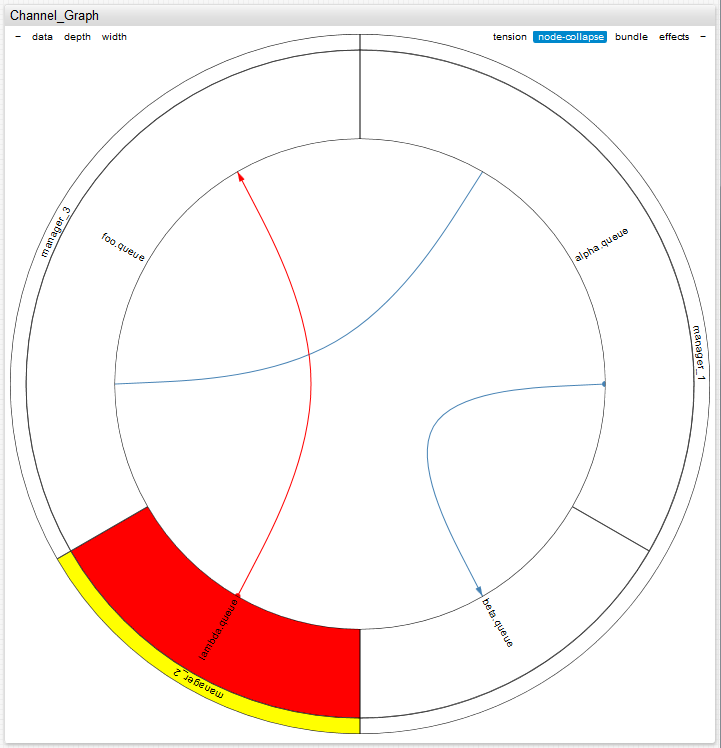#### Thresholds

Thresholds for time series tied to vertices or edges are set using the link-threshold or node-threshold parameters under the [widget] tag. In this case, color and width parameters are applied to the edge and vertices based on link-widths and link-colors (node-radiuses and node-colors) under the [widget] tag.

Styles of alert-expression are superimposed over the styles defined by thresholds.

##### Example:
```[widget] mode = hierarchy type = graph title = Channel_Graph bundle = false data = true depth = 3 max-ring-width = .3 link-thresholds = 1000 link-thresholds = Math.max(percentile(25), 50000) link-thresholds = percentile(90) link-widths = 1, 1, 2, 3 link-colors = blue, green, orange, red [node] id = manager_1 [node] id = manager_2 alert-style = fill: yellow [node] id = manager_3   [node] id = alpha.queue parent = manager_1   [node] id = beta.queue parent = manager_1   [node] id = lambda.queue parent = manager_2   [node] id = foo.queue parent = manager_3   [link] nodes = alpha.queue - foo.queue   [link] nodes = lambda.queue -> foo.queue   [link] nodes = beta.queue <- alpha.queue   [series] entity = gr02 metric = message_count links = lambda.queue - foo.queue   [tag] name = channel_name value = THE.BEST.CH   [series] entity = gr02 metric = message_count links = alpha.queue - foo.queue   [tag] name = channel_name value = ALPHA.FOO.CH   [series] entity = gr02 metric = message_count links = alpha.queue - beta.queue   nodes = beta.queue   [tag] name = channel_name value = ALPHA.BETA.CH```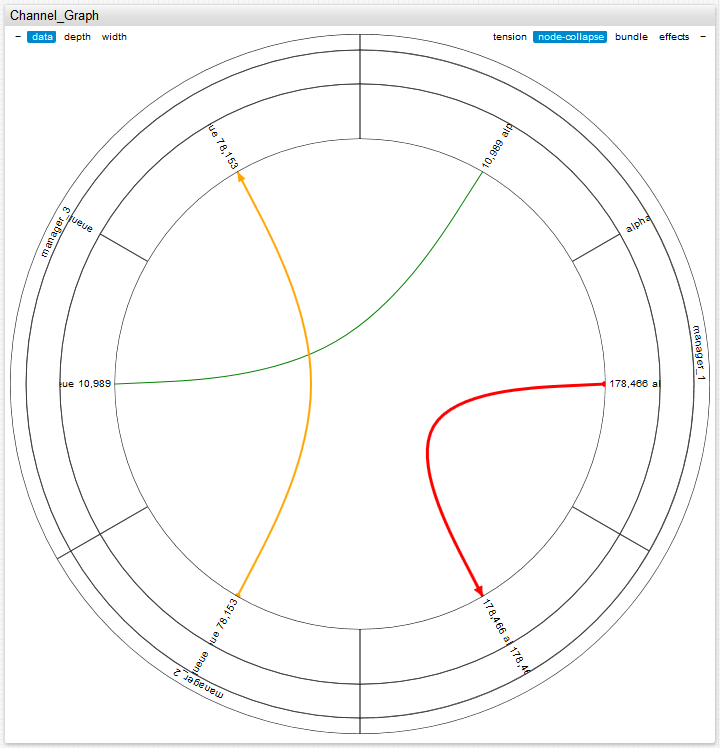#### Settings

##### [widget] settings:
SettingDescription
`mode`Determines the overall look of the widget, possible values: hierarchy or non-hierarchy (non-hierarchy by default).
`layout`Form of the widget in non-hierarchy mode, possible values: circle or rectangle (circle by default).
`depth`Depth of the displayed vertex hierarchy from 1 to the maximum depth of the vertex hierarchy plus 1 (final level corresponds to edges) (maximal dept of the hierarchy be default).
`tension`The degree curvature of the edges; corresponds to the parameter C in the formula for the cardinal spline; varies from 0 to 1 (0 corresponds to straight edges) (0.9 be default).
`bundled`Hierarchical contraction of edges (Danny Holten algorithm) (true by default).
`effects`Animation when changing the geometry of the graph (false by default).
`duration`The duration of the transaction when changing the geometry of the graph in milliseconds (1000 by default).
`min-ring-width`The minimum allowed width of the vertex ring. The value is from 0 to a maximum permissible value of the ring width (0.3 by default).
`max-ring-width`The maximum allowed width of the vertex ring from the current minimal value of the ring width to (0.5 by default).
`arrows`Arrows on the directed edges (false by default).
`node-connect`Connect vertices on the perimeter (true by default).
`data`Information about the last time series value next to the image of the corresponding vertex (false by default).
`node-collapse`Collapse vertex sectors when clicked (true by default).
`node-alert-style`Style of vertices, in the event of an alert-expression being triggered tied to the time series.
`link-alert-style`Style of edges in the event of an alert-expression being triggered and tied to the time series.
`link-thresholds`Threshold for time series attached to the edges. Can use javascript and percentile functions.
`link-colors`Color to indicate the threshold of time series. It is attached to the edges and separated by commas.
`link-widths`Width to indicate the thresholds of the time series tied to the edges and separated by commas or spaces.
`node-thresholds`Threshold for time series tied to vertices. Can use javascript, percentile functions, or can be set as an array.
`node-colors`Color to indicate the threshold of time series that are bound to vertices and separated by commas.
`node-radiuses`Radii of the lighting for the display of the time series thresholds linked to the vertices and separated by commas or spaces.
`node-radius`Radius of the vertex in pixels (not valid in hierarchy mode).
`padding`Offset from the edge of the container in pixels.
`link-animate`Animation of directed vertices (false by default).
`disconnected-node-display`Show vertices without edges, values: true or false (true by default).
`node-labels`Display vertex labels; possible values: true or false.
`link-labels`Display edge labels; possible values: true or false.
`link-data`Series last value next to the link-label.
`link-color-range`Color scheme for link-threshold.
`link-label-zoom-threshold`Parameter value is a value of scale when labels appear based on edge threshold; value <1 - labels disappear on zoom out, value >1 – labels appear on zoom in.
`node-label-zoom-threshold`Parameter value is a value of scale when labels appear based on vertex threshold; value <1 - labels disappear on zoom out, value >1 – labels appear on zoom in.
`auto-padding`Add padding if labels overflow container, possible values: true or false (true by default).
`link-width-order`Sort links according to their width, possible values: top, bottom or undefined (undefined by default).
##### Vertex settings, [node]:
SettingDescription
`id`Unique name of the vertex, a required setting.
`parent`id of parent vertex.
`series`Alias of the time series associated with the vertex.
`alert-style`Vertex style, in the event of an alert-expression for the associated time series triggering.
`tooltip`Add custom string to tooltip.
`label`Text on label; element id by default.

You can use `[properties]` to specify any values divided by an equal sign (if the value contains an equal sign `=` or `\`, then they should be written with a backslash: `\=` or `\\`).

Chart Lab Example for `[properties]`View

SettingDescription
`id`Unique edge name.
`nodes`Vertices connected by an edge; id of vertices, separated by a comma or a hyphen for undirected edges, -} or {- for directed edges; if vertex id contains a hyphen, it must be shielded using the “” symbol.
`series`Alias of time series associated with the edge.
`alert-style`Edge style, in the event of an alert-expression of the associated time series triggering.
`tooltip`Add custom string to tooltip.
`label`Text on label; element id by default.

You can use `[properties]` to specify any values divided by an equal sign (if the value contains an equal sign `=` or `\`, then they should be written with a backslash: `\=` or `\\`).

Chart Lab Example for `[properties]`View

##### [series] settings, associating time series to the graph:
SettingDescription
`nodes`List of vertices associated with this series; when the vertex id contains a hyphen, it must be shielded with symbol.
`links`List of edges associated with this series is defined by an id or edge id of the corresponding vertices with a hyphen; when the edge id contains a hyphen, it must be shielded with a symbol.
`link-alerts-style`Edge style to which a time series is bound in the event of an alert-expression being triggered.
`node-alert-style`Vertex style to which a time series is bound in the event of an alert-expression being triggered.

It is possible to create an expanded mouse over tool-tip containing contextual information. Settings are used under the [series] tag.  It is possible to prescribe the [properties] tag which specifies any values divided by an equal sign (if the value contains an equal sign = or \, then they should be written with a backslash – \ = \\). They are displayed in a table within the tool-tip. Also in these tags, you can use the context setting value which is displayed in the tool-tip before the [properties] tool-tip table. Within the context setting, you can create a table using standard html parameters: table class, tbody, tr, td.

#### Controls

The graph appearance can be adjusted by the controls located in the top corners of the widget: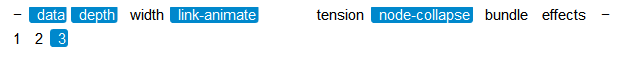SettingDescription
`data`Show or hide information about the last value of a time series next to the corresponding image of the vertices (by default, the information is hidden).
`depth`Set the depth of the displayed vertex hierarchy; allows a value of 1 to a maximum height of the vertex tree plus 1 (the last level corresponds to the edges); by default, the depth is equal to the height of the vertex tree displayed.
`width`Maximum and minimum allowed width of the vertex ring is the percent from the radius of the circle (by default, a minimum 30%, maximum 50%).
`tension`The degree of edge curvature. Corresponds to the parameter C in the formula for the cardinal spline. Varies from 0 to 1 (0 corresponds to direct edges) (default value is 0.9).
`bundle`Hierarchical contraction of edges (the Danny Holten algorithm); used by default.
`effects`Animation when changing the geometry of the graph; used by default.
`node-collapse`Collapse sector peaks when clicked; true by default.
`link-animate`Animation of directed edges; false by default.